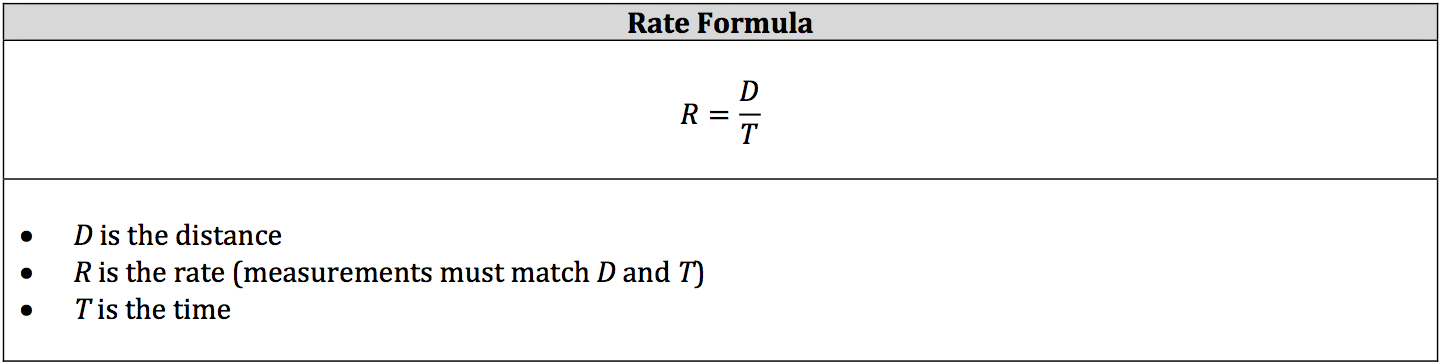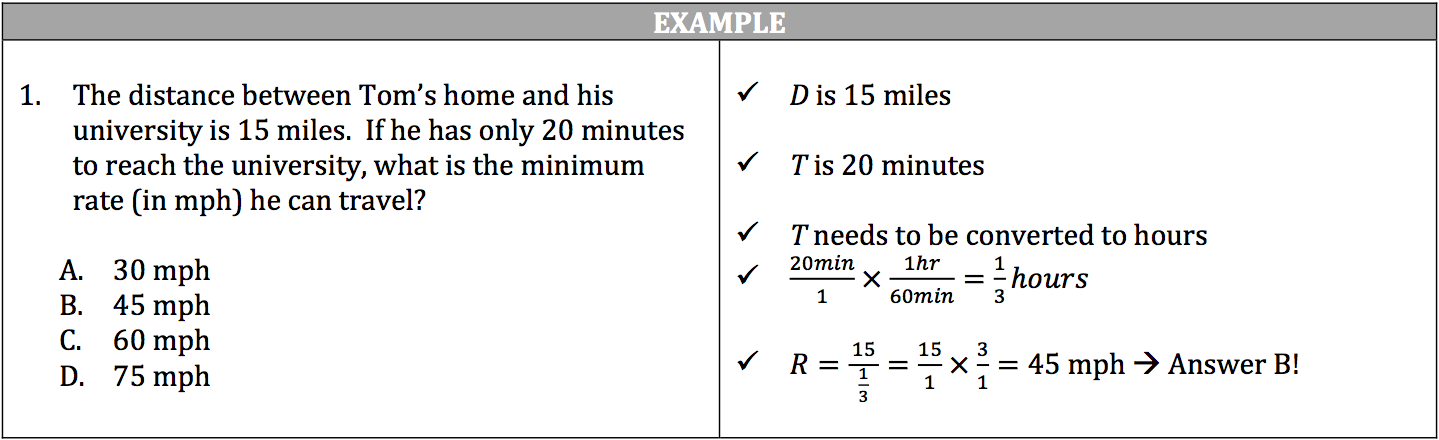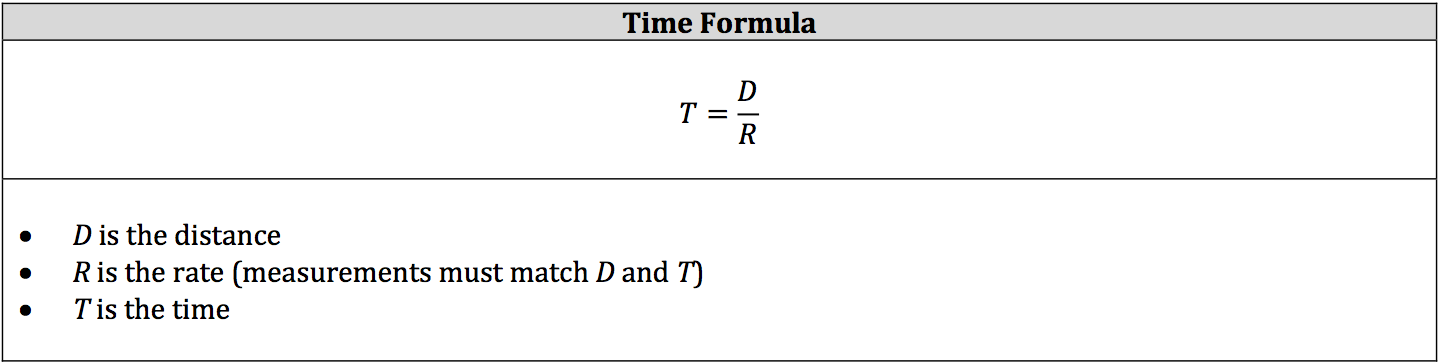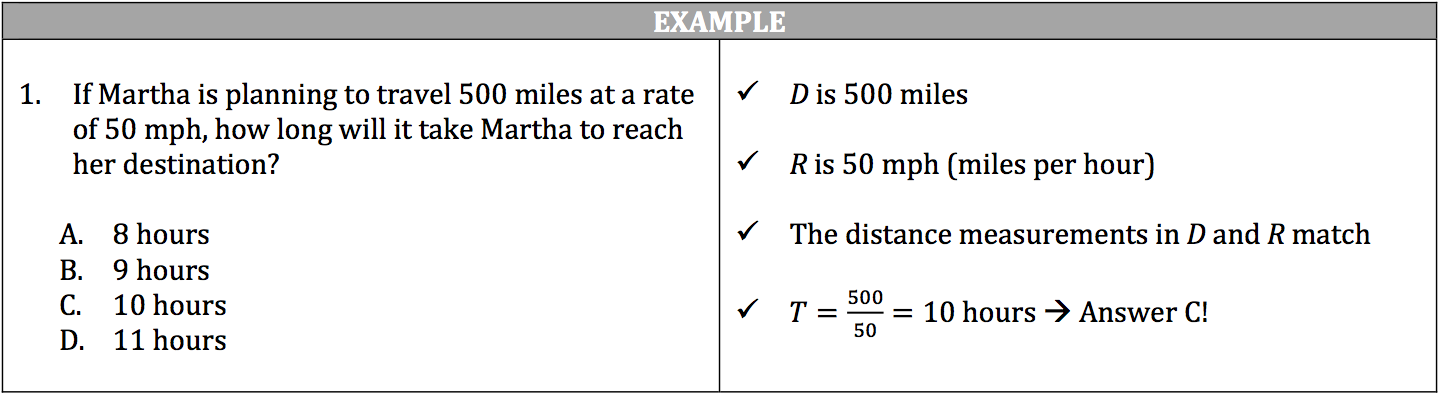You’ve ridden in a car enough times to know it travels a specific rate, such as miles per hour, to reach its destination. You’ve also seen the speed limit signs designating the maximum rate allowed for a particular stretch of road. If you look up directions to a place you want to go, then you’ll find the distance between your current location and your destination and the time it will take you to get there. These calculations are based on the simple formulas for distance, rate, and time.

### Distance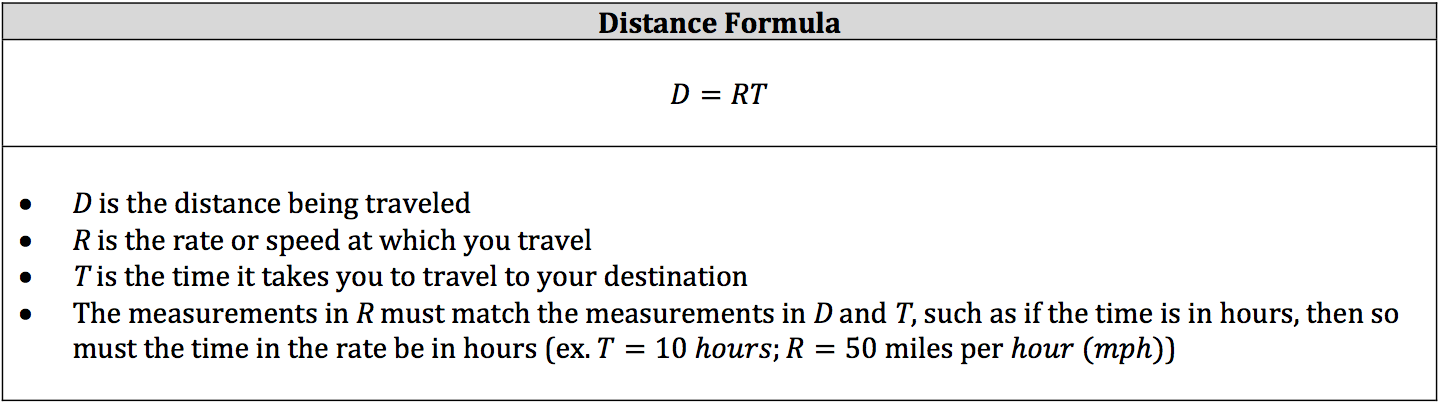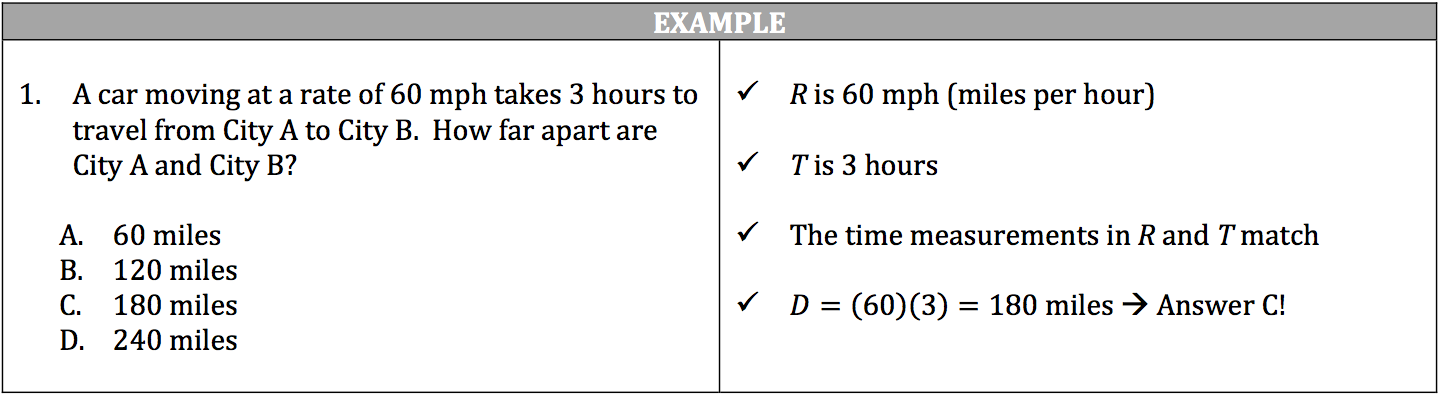### Rate and Time

The formulas for rate and time are actually variations of the distance formula. If you have a hard time remembering the other two formulas, simply remember the distance formula. Since the distance will be known when finding the rate or the time, the other known value will divide into the distance, leaving the unknown by itself on one side of the equation.